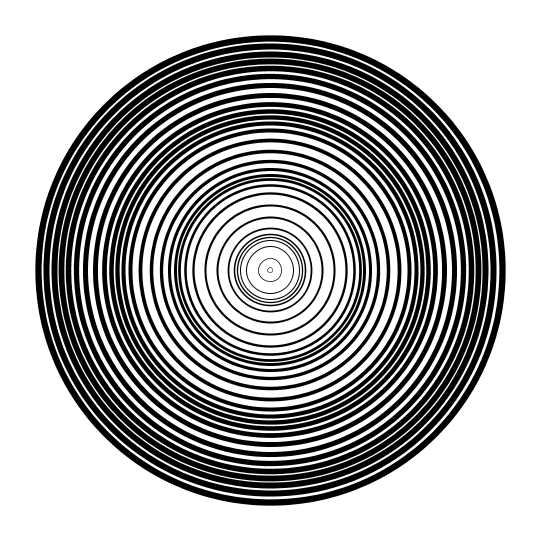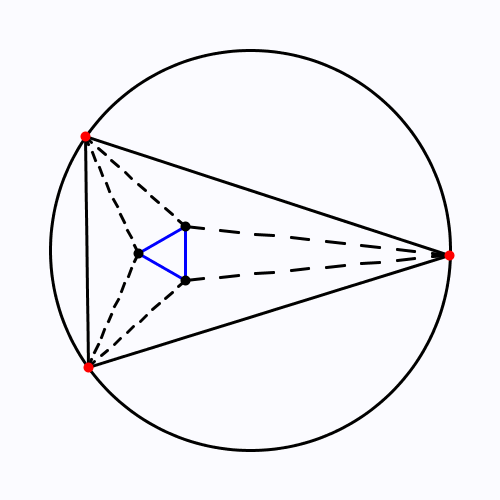PhotoPhoto

Triangulating a circle. How to do a drawing li…Triangulating a circle.

How to do a drawing like this? Start with a circle and divide it into n equal parts. I choose n=100 for this drawing. There is an easy formula for this, the i-th point is (radius*cos(i*2*PI/n),

radius*sin(i*2*PI/n)). Then for each i connect the i-th point to the point  (radius*cos(PI-2*i*2*PI/n), radius*sin(PI-2*i*2*PI/n)). This might not be one of the original points, but it is on the circle. And that is it 🙂

Why is this mathematically interesting?  This way we get a set of nonparallel  lines such that there are a lot of triple intersections between them.

Triangulating a circle. How to do a drawing li…Triangulating a circle.

How to do a drawing like this? Start with a circle and divide it into n equal parts. I choose n=100 for this drawing. There is an easy formula for this, the i-th point is (radius*cos(i*2*PI/n),

radius*sin(i*2*PI/n)). Then for each i connect the i-th point to the point  (radius*cos(PI-2*i*2*PI/n), radius*sin(PI-2*i*2*PI/n)). This might not be one of the original points, but it is on the circle. And that is it 🙂

Why is this mathematically interesting?  This way we get a set of nonparallel  lines such that there are a lot of triple intersections between them.

Moons.I have reached 10000 followers! Thank you for the…Moons.

I have reached 10000 followers! Thank you for the support!!!

Limiting proof of area of a circle.Limiting proof of area of a circle.

thousandmaths: szimmetria-airtemmizs: We hav…We have three colored segment in this animation. Surprisingly the length of the longest one is always the sum of the length of the two smaller ones.

This is actually a very special case of Ptolemy’s theorem. The theorem gives a connection between the sides and the diagonals of a cyclic quadrilateral. In this case the length of the dashed lines is equal so the theorem can be simplified to the statement above.

[ Holy cow, this blog is fantastic. Folks, do yourself a favor and give it a looksie. ]

——

Reducing to Ptolemy’s Theorem…

It is fairly easy to derive this result if you already know Ptolemy’s theorem, and I’d like to go through that calculation. I will state the theorem in full generality but I will only write down the equations for the “very special case” considered in the animation.

For any four-sided shape inscribed inside a circle:

The product of the two diagonals…

$$b \times s$$

…is equal to the sum of…

$$b \times s = (?) + (?)$$

… the products of…

$$b \times s = (? \times ?) + (? \times ?)$$

… the pairs of opposite sides:

$$b \times s = (a \times s) + (c \times s)$$

Or, written in more usual algebraic shorthand: $bs = as+cs$.

Since $s$ is not zero (otherwise our ‘triangle’ would look pretty silly…), we can divide by $s$ to get $b=a+c$. And this is, indeed, an algebraic rewriting of “the length of the longest side is the sum of the lengths of the two smaller ones.”

——

… and a (much) more general lesson

Notice that for quadrilaterals not created via an equilateral triangle, each of the three instances of $s$ might actually be different numbers, which is unfortunate in that instead of taking a sum $b=a+c$ you have to take a weighted sum $b=w_1a+w_2c$. Actually it’s worse than that: in general these weights will change depending on which arc the extra point is in, and basically it’s a huge mess and you don’t want to phrase the theorem this way.

But in the case of an equilateral triangle, we suddenly have this really nice and intuitive way of thinking about the situation.

This sort of thing happens all the time in math: we can state a reasonable-sounding fact in rather technical language, but when we restrict our attention to special cases we free ourselves up to work in an qualitatively, substantially simpler context. Here, for instance, we were able to eliminate algebra entirely from the picture. Long-time readers of my blog may also consider the example of Coxeter groups: in general these have to be phrased as group presentations, but in “Type A” we suddenly don’t even need groups at all, and just think about permutations.

This is obviously a very useful feature of the subject in terms of communication, since if you can find such a reduction, you can make an otherwise technical result much easier to talk about. But it’s a somewhat annoying feature for the working mathematician because it means that the tools you want to use to analyze a situation can look radically different from the situation itself. The easiest way to go about proving the result claimed in the animation seems like it should have something to do with the geometry of circles and triangles. But in hindsight, it turns out to follow from a standard fact in the geometry of quadrilaterals

I’ll finish the post with two different conclusions:

(1) The above is a particularly tame example, as math goes: at least we are still in geometry! Oftentimes you have to be willing to travel much further to find these “generalized explanations”, and there is never any real guarantee that you will find one. Therefore, this phenomenon is a partial justification for the Vakil approach to learning mathematics, or Tao’s advice on giving talks, or Lady’s method for finding ideas.

(2) This phenomenon also, I think, sheds some light on why mathematicians have to do actual work to determine whether an unsolicited paper is the work of a crank. Probably the most famous example of people writing about their efforts in this regard is Hardy’s comment “The first question was whether I could recognize anything” from Ramanujan’s initial letter. Things like Aaronson’s list exist because they providing heuristic shortcuts for safely dismissing bad math without doing the onerous work required to find good math.

thousandmaths: szimmetria-airtemmizs: We have three colored…We have three colored segment in this animation. Surprisingly the length of the longest one is always the sum of the length of the two smaller ones.

This is actually a very special case of Ptolemy’s theorem. The theorem gives a connection between the sides and the diagonals of a cyclic quadrilateral. In this case the length of the dashed lines is equal so the theorem can be simplified to the statement above.

[ Holy cow, this blog is fantastic. Folks, do yourself a favor and give it a looksie. ]

——

Reducing to Ptolemy’s Theorem…

It is fairly easy to derive this result if you already know Ptolemy’s theorem, and I’d like to go through that calculation. I will state the theorem in full generality but I will only write down the equations for the “very special case” considered in the animation.

For any four-sided shape inscribed inside a circle:

The product of the two diagonals…

$$b \times s$$

…is equal to the sum of…

$$b \times s = (?) + (?)$$

… the products of…

$$b \times s = (? \times ?) + (? \times ?)$$

… the pairs of opposite sides:

$$b \times s = (a \times s) + (c \times s)$$

Or, written in more usual algebraic shorthand: $bs = as+cs$.

Since $s$ is not zero (otherwise our ‘triangle’ would look pretty silly…), we can divide by $s$ to get $b=a+c$. And this is, indeed, an algebraic rewriting of “the length of the longest side is the sum of the lengths of the two smaller ones.”

——

… and a (much) more general lesson

Notice that for quadrilaterals not created via an equilateral triangle, each of the three instances of $s$ might actually be different numbers, which is unfortunate in that instead of taking a sum $b=a+c$ you have to take a weighted sum $b=w_1a+w_2c$. Actually it’s worse than that: in general these weights will change depending on which arc the extra point is in, and basically it’s a huge mess and you don’t want to phrase the theorem this way.

But in the case of an equilateral triangle, we suddenly have this really nice and intuitive way of thinking about the situation.

This sort of thing happens all the time in math: we can state a reasonable-sounding fact in rather technical language, but when we restrict our attention to special cases we free ourselves up to work in an qualitatively, substantially simpler context. Here, for instance, we were able to eliminate algebra entirely from the picture. Long-time readers of my blog may also consider the example of Coxeter groups: in general these have to be phrased as group presentations, but in “Type A” we suddenly don’t even need groups at all, and just think about permutations.

This is obviously a very useful feature of the subject in terms of communication, since if you can find such a reduction, you can make an otherwise technical result much easier to talk about. But it’s a somewhat annoying feature for the working mathematician because it means that the tools you want to use to analyze a situation can look radically different from the situation itself. The easiest way to go about proving the result claimed in the animation seems like it should have something to do with the geometry of circles and triangles. But in hindsight, it turns out to follow from a standard fact in the geometry of quadrilaterals

I’ll finish the post with two different conclusions:

(1) The above is a particularly tame example, as math goes: at least we are still in geometry! Oftentimes you have to be willing to travel much further to find these “generalized explanations”, and there is never any real guarantee that you will find one. Therefore, this phenomenon is a partial justification for the Vakil approach to learning mathematics, or Tao’s advice on giving talks, or Lady’s method for finding ideas.

(2) This phenomenon also, I think, sheds some light on why mathematicians have to do actual work to determine whether an unsolicited paper is the work of a crank. Probably the most famous example of people writing about their efforts in this regard is Hardy’s comment “The first question was whether I could recognize anything” from Ramanujan’s initial letter. Things like Aaronson’s list exist because they providing heuristic shortcuts for safely dismissing bad math without doing the onerous work required to find good math.Circular waves.

szimmetria-airtemmizs: Morley theorem 2.  The …Morley theorem 2.

The dashed lines are angle trisectors, so they divide the angles of the triangle into three equal parts. The blue triangle is equilateral, which means that each blue segment has the same length.

szimmetria-airtemmizs: Morley theorem 2.  The dashed lines are…Morley theorem 2.

The dashed lines are angle trisectors, so they divide the angles of the triangle into three equal parts. The blue triangle is equilateral, which means that each blue segment has the same length.